# Mathematics Assignment

ASSIGNMENT 1
Mathematics Assignment
Student Name
Institutional Affiliation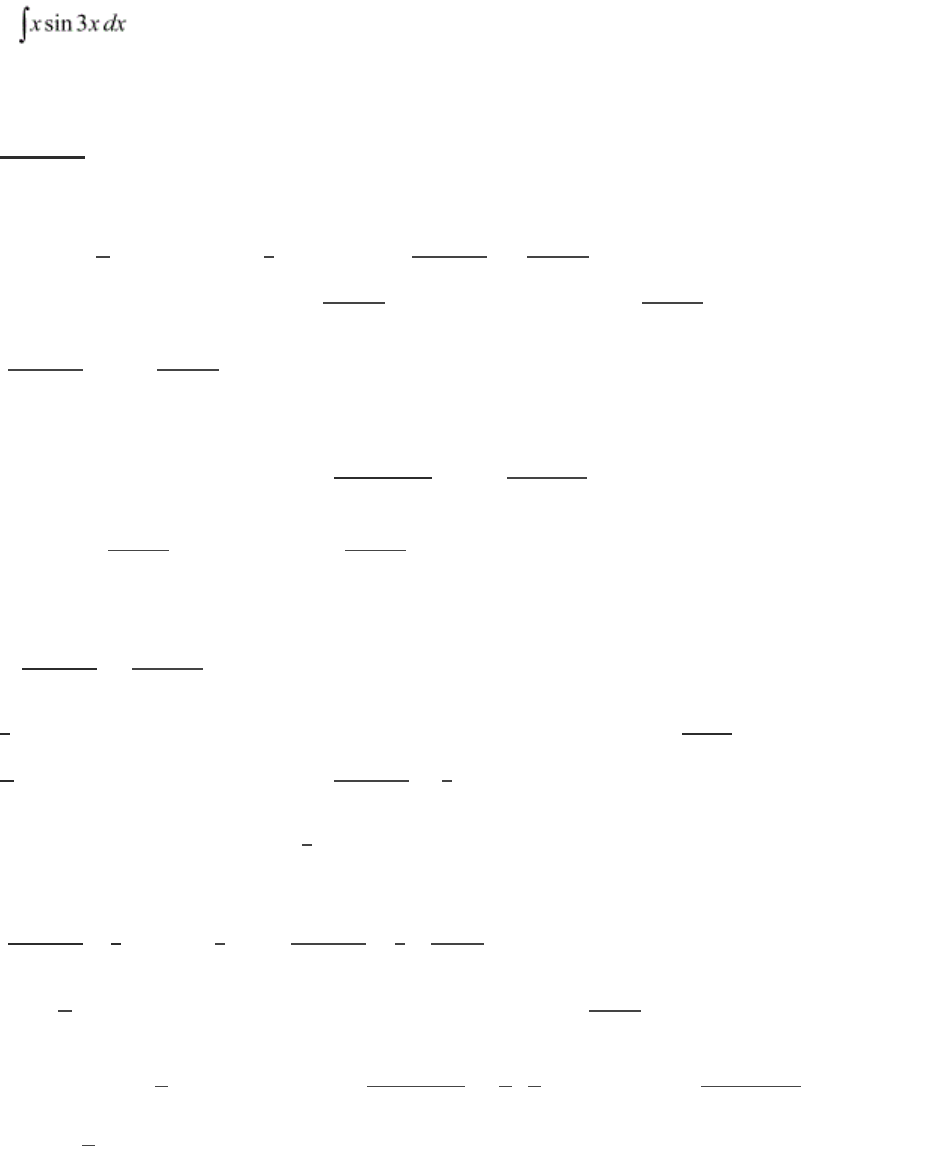MATHEMATICS ASSIGNMENT 2
Provide solution to the following questions:
Question 1. Evaluate the following:
Solution
Integrating by parts, we apply the formula ∫ udv = uv - ∫ vdu such that u = x and dv = sin (3x).
Thus x(

cos (3x)) - -
cos (3x) dx

- -

. However, since -1 is a constant
with respect to x, we integrate

with respect to x to get -


-




Multiplying -1 by -1 we get 1, thus


 


Multiply

 by 1, to get


Thus





is a constant with respect to x in both equations, thus, the integral of

with respect to x is




 



.
Let u = 3x, and du = 3dx, thus
 . The equation can then be rewritten using u and du
such that,
-







Since





 




 



 
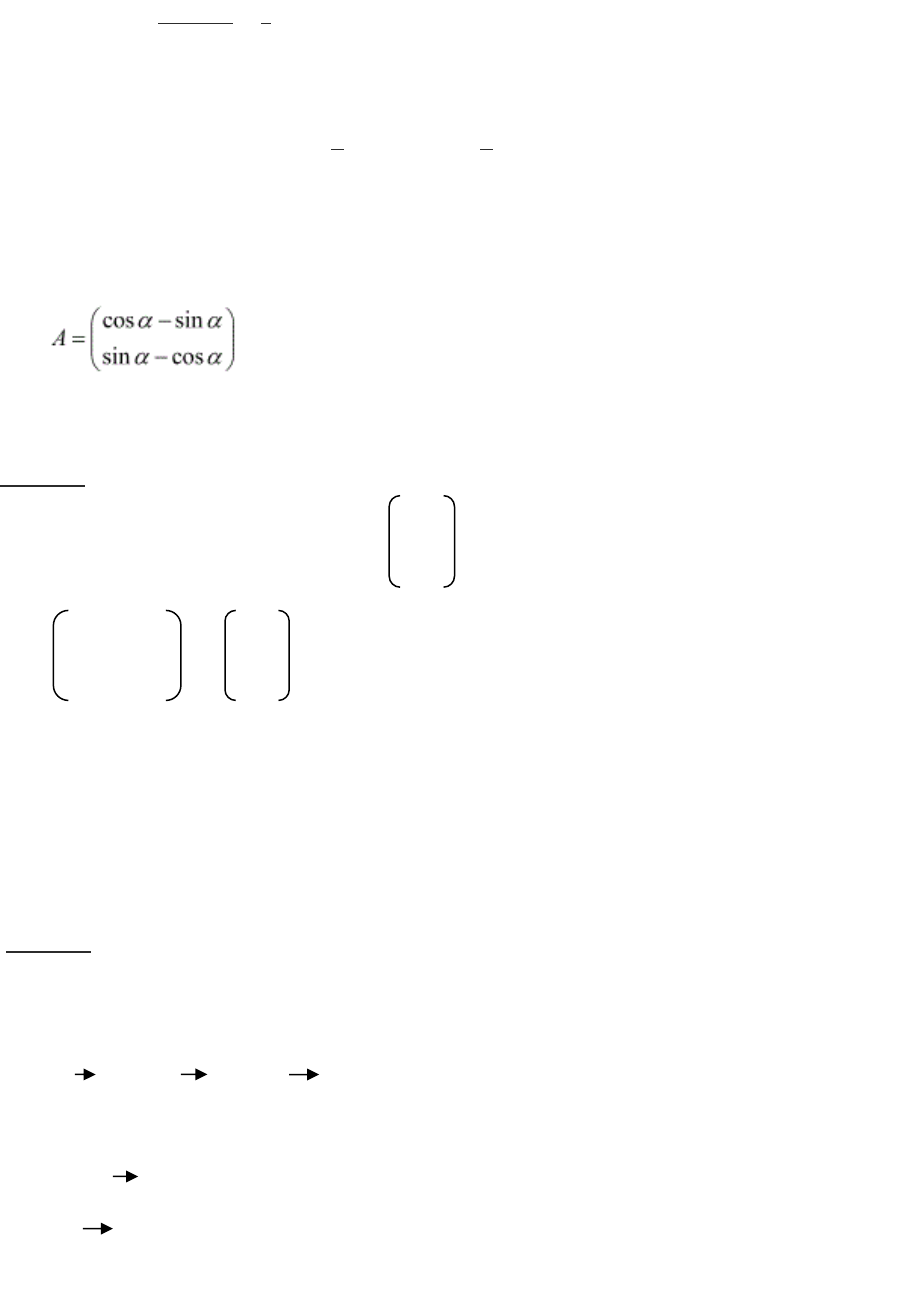MATHEMATICS ASSIGNMENT 3
This becomes
 

  
Simplifying the expression, the answer becomes;




 
2. If , then for what value of α is A an identity matrix?
Solution
identity matrix of A is given by AI = 1 0
0 1
Thus Cosα -sinα = 1 0 Thus, cos α = 1, –sin = 0, sin α = 0, and cos α = 1
Sinα cosα 0 1
Therefore the value of α in the identity matrix A is 0 or 1
3. The line y = mx + 1 is a tangent to the curve y
2
= 4x .Find the value of m.
Solution
If y = mx + 1 is the tangent to curve y
2
= 4x, then the derivative of the curve equals the slope of
the line y = mx + 1.
y
2
= 4x 2yy’ = 4 y= 2/y m = 2/y
Solving the equation simultaneously,
Y= mx + 1 x = (y-1)/m
Y
2
= 4x x = y
2
/4
x=xMATHEMATICS ASSIGNMENT 4
(y-1)/m = y²/4
4(y-1) = y²m
4(y-1) = y²(2/y) substituting the value of m
4y - 4 = 2y
2y = 4
y = 2
Therefore
m = 2/y = 2/2 = 1
The value of m is 1
4. Solve the following differential equation: (x
2
y
2
) dx + 2 xy dy = 0.
Solution
From the equation = (x
2
y
2
) dx + 2 xy dy = 0.
y' + p(x)y=q(x)yα.
Substitute 2xy dy on both sides to get 2xy dy=(x
2
+y
2
)dx, then divide by dx to
get 2xydydx=x
2
+y
2
.
Dividing by 2xy to get dydx=x
2
y−1+12xy.
Putting this in the form y'+p(x)y=q(x)yα as follows: y'−12xy=x
2
y−1.
Substituting v=y1−α
ddx[v=y1−α]dvdx=(1−α)y−αdydx.
So dydx=11−αyαdvdx.
Making y the subject,
Since v=y1−α, v=y1y−α.
So y=vyα
Sub-backing into the form y'+p(x)y=q(x)yα we get 11−αyαdvdx+p(x)vyα=q(x)yα.
To make it a linear equation, we divide through to get; dvdx+(1−α)p(x)v=(1−α)q(x).MATHEMATICS ASSIGNMENT 5
Having formed a first order linear differential equation, we then solve using an integrating factor,
Looking back at the actual problem,
We have y'−12xy=x2y−1.
α=−1, substituting; v=y1−(−1)v=y2.
Since v=y2, then dvdx=2ydydx, so dydx=12y−1dvdx.
We know that y=vy−1.
Considering that y'−12xy=x2y−1 we plug in the equation such that
12y−1dvdx−12xy−1v=x2y−1.
Thus we get,12dvdx−12xv=x2.
divide by 12 to standardize the linear equation.
v'−1xv=x.
solving;
I(x)=e∫p(x)dx, where p(x)=−1x.
∫p(x)dx=∫−1xdx=−lnx.
e−lnx=1x.
Multiply by 1x to get 1xv'−1xv=1.
ddx[1xv]=1, so 1xv=∫1dx=x+C.
Solve for v to get v=x
2
+Cx.
But v=y
2
.
Thus,
Y
2
=x
2
+Cx.
2
=x
2
+Cx.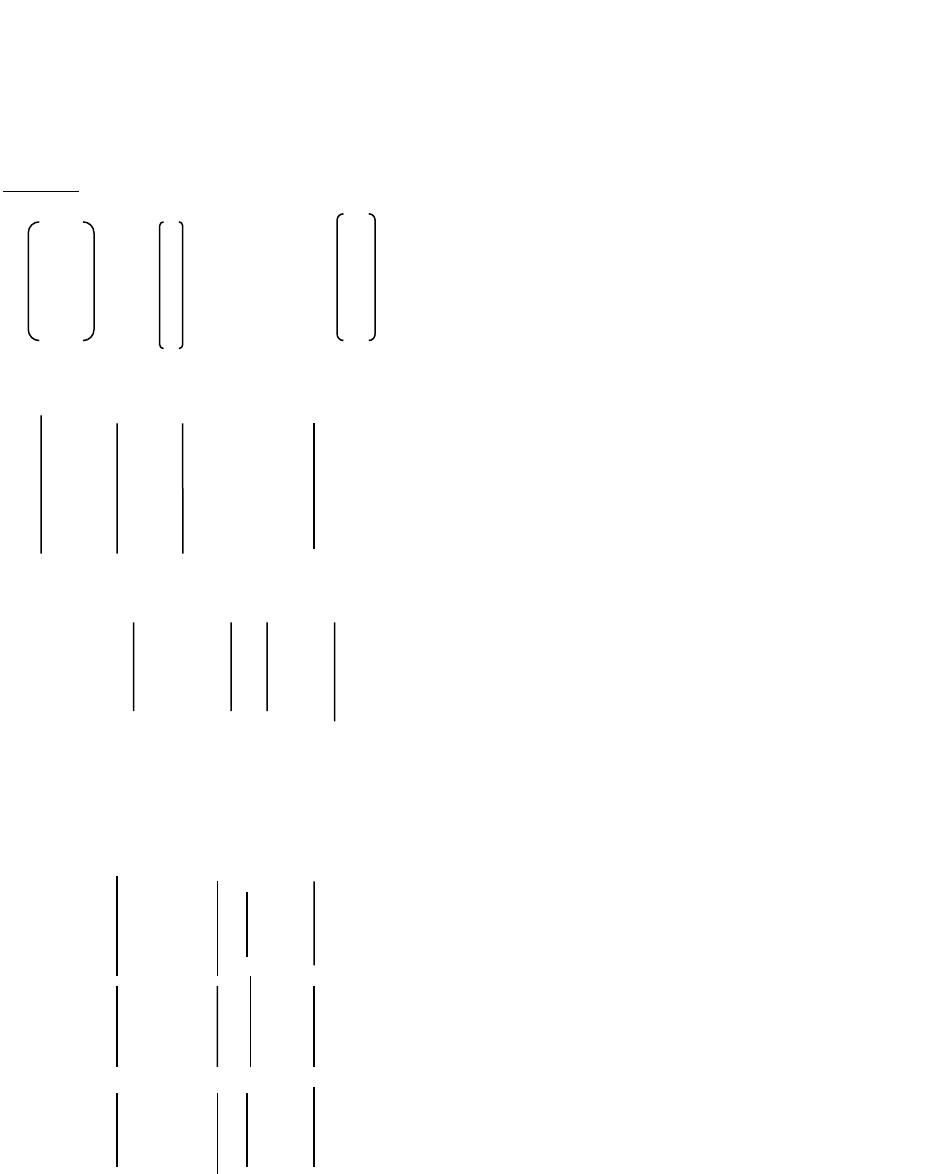MATHEMATICS ASSIGNMENT 6
5. Solve system of linear equations, using matrix method.
x y +2z =7
3x +4y −5z =−5
2x y + 3z = 12
Solution
1 -1 2 x 7
A= 3 4 -5 X = y B = -5
2 -1 3 z 12
1 -1 2 a11 a12 a13
2 4 -5 = a21 a22 a23
2 -1 3 a31 a32 a33
A11 = (-1)
1+1
a22 a23 = 4 -5
a32 a33 -1 3 = {4x3-(-1x5)} =7
A12 = (-1)
1+2
a21 a23 3 -5
a31 a33 = 2 3 = -{3x3-(2x-5)} = 19
A13 = (-1)
1+3
a21 a22 3 4
a31 a32 = 2 -1 = {3x-1-(2x4)} = -11
A21 = (-1)
2+1
a12 a13 -1 2
a32 a33 = -1 3 = {-1x3-(-1x2)} =1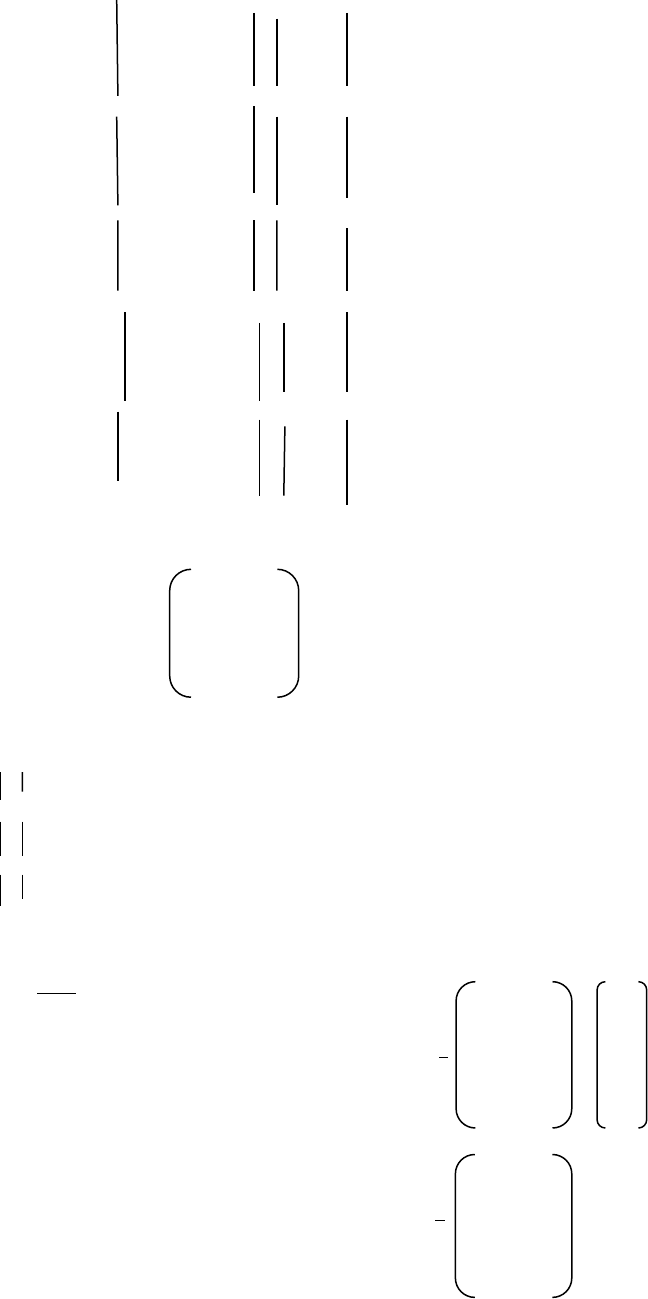MATHEMATICS ASSIGNMENT 7
A22 = (-1)
2+2
a11 a13 1 2
a31 a33 = 2 3 ={1x3- (2x2)} =-1
A23 = (-1)
2+3
a11 a12 = 1 -1
a31 a32 2 -1 = {1x-1-(2x2)} =-1
A31 = (-1)
3+1
a12 a13 = -1 2
a22 a23 4 -5 = {-1x-5-(4x2)} = -3
A32 = (-1)
3+2
a11 a13 1 2
a21 a23 = 3 -5 = -{1x-5-(3x2) = 11
A33 = (-1)
3+3
a11 a12 1 -1
a21 a22 = 3 4 = {1x4 (3x-1)} = 7
7 1 -3
-11 -1 7
A : a11 x A11 + a12 x A12 + a13 x A13
A : 1x7 + -1 x -19 + 2 x -11
A = 4
Using the formula
X =

7 1 -3 7
we plug the value of
to get X =
-19 -1 11 -5
-11 -1 7 12
49 -5 -36
X =
-133+ 5+132
-77+5+84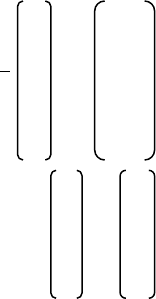MATHEMATICS ASSIGNMENT 8
8 8/4
X =
4 = 4/4
12 12/4
x 2
thus, y = 1
z 3
Thus X= 2, Y= 1, and Z = 3

-+
550 words

#### Our customers say##### Jeff Curtis
USA, Student

"I'm fully satisfied with the essay I've just received. When I read it, I felt like it was exactly what I wanted to say, but couldn’t find the necessary words. Thank you!"##### Ian McGregor
UK, Student

"I don’t know what I would do without your assistance! With your help, I met my deadline just in time and the work was very professional. I will be back in several days with another assignment!"##### Shannon Williams

"It was the perfect experience! I enjoyed working with my writer, he delivered my work on time and followed all the guidelines about the referencing and contents."

• 5-paragraph Essay
• Annotated Bibliography
• Argumentative Essay
• Article Review
• Assignment
• Biography
• Book/Movie Review
• Case Study
• Cause and Effect Essay
• Classification Essay
• Comparison Essay
• Coursework
• Creative Writing
• Critical Thinking/Review
• Deductive Essay
• Definition Essay
• Essay (Any Type)
• Exploratory Essay
• Expository Essay
• Informal Essay
• Literature Essay
• Multiple Choice Question
• Narrative Essay
• Personal Essay
• Persuasive Essay
• Powerpoint Presentation
• Reflective Writing
• Research Essay
• Response Essay
• Scholarship Essay
• Term Paper
We use cookies to provide you with the best possible experience. By using this website you are accepting the use of cookies mentioned in our Privacy Policy.